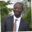Related Tags

java
communitycreator

# What are formal and actual parameters in Java?Abel Lifaefi Mbula

A parameter is a piece of information that is passed into a method to be used by the method’s internal computations. Parameters are used to customize the behavior of a method in order to adapt it to a particular situation.

Let’s look at an example.

public static boolean isEven(int number) {
return number % 2 == 0;
}


In the code above, we create a static method, isEven, that accepts one parameter of type integer.

Now that we’ve defined isEven, let’s call it in the main method.

public static void main(String[] args) {
// Print even or odd number from 0 to 15
int limit = 15;
int count = 0;

while (count < limit) {

if (isEven(count)) // count is the argument for isEven method
System.out.println(count + " is an even mumber");
else
System.out.println(count + " is an odd mumber");

count++;

}
}


We call isEven() with the count parameter. We define our method with int number and call it with count.

Depending on whether a parameter is used in the method definition or the method call, it is called a formal/dummy parameter or actual parameter/argument. The formal parameter is a simple identifier, the name, and the actual parameter the value (of the same type as the identifier). If we consider the example above, number is the formal parameter and count is the argument.

Let’s put it all together and execute the program.

public class EvenOdd {

public static void main(String[] args) {
// Print even or odd number from 0 to 15
int limit = 15;
int count = 0;

while (count < limit) {

if (isEven(count)) // count is the argument for isEven method
System.out.println(count + " is an even mumber.");
else
System.out.println(count + " is an odd mumber.");

count++;

}
}

public static boolean isEven(int number) { // number is a formal parameter of type int
return number % 2 == 0;
}

}

RELATED TAGS

java
communitycreator

CONTRIBUTORAbel Lifaefi Mbula
RELATED COURSES

View all Courses

Keep Exploring

Learn in-demand tech skills in half the time